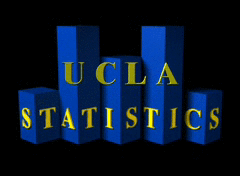## Additional SOCR ResourcesAll of the following links require these two freely available software tools: JavaScript and Java, to be installed on your local machine.
 High-Precision Distribution Calculators Conceptual Demo Applets Tables Online Real-Time Statistics Data Analysis Function and Image-Processing Tools Other Online Compute Resources Other Interesting Java Applets JavaScript HTML5 Apps

 High-Precision Distribution Calculators Conceptual Demo Applets Interactive Normal Curve (applet). Galton's Board (Quincunx) (illustration of CLT) Sampling Distribution Applet (illustration of CLT) Simple linear regression (applet) Normal Approximation to Poisson Distribution Dice Applet Confidence Intervals Applet Tables How to Choose the Right Statistical Test? T-table, Normal Z-table and Chi-Square table and F tables Wilcoxon Rank-Sum table Online Statistics Packages for Real-Time Data Analysis ANOVA (Single and Multi-Way Analysis of Variance - Java Applet). Other statistical tests (e.g., T-tests from raw data or summaries, Chi-Square tests, etc.) Function and Image-Processing Tools Other Online Probability & Statistics Computational Resources Other Interesting Java Applets JavaScript HTML5 Apps